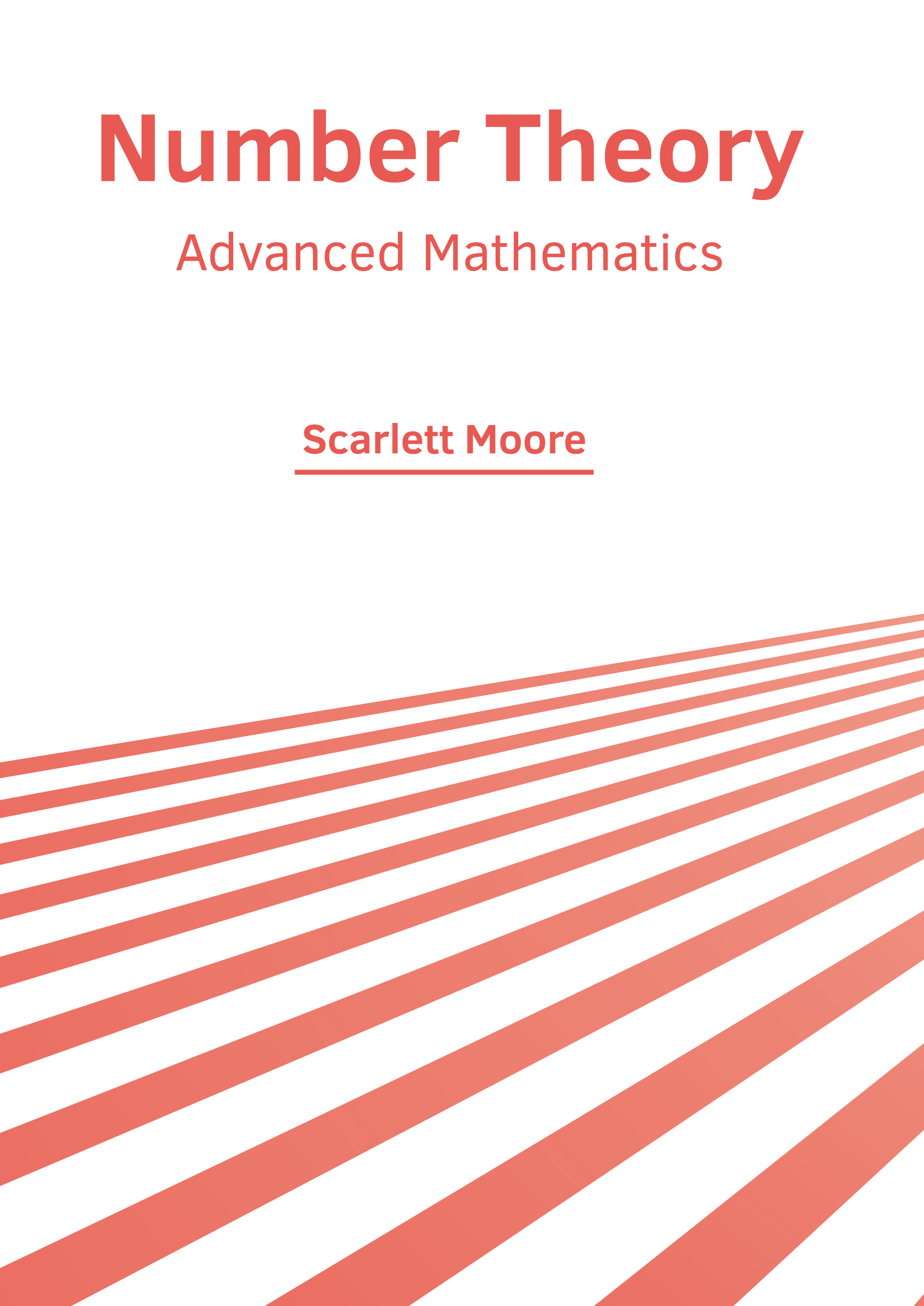BROWSE BY

#### Author

##### Home / Books / Number Theory: Advanced Mathematics9781647253516

Author

Scarlett Moore

##### Publisher

NY Research Press

English

2021

##### Category

Mathematics - Mathematics

\$ 154.95

##### Description

The branch of pure mathematics devoted primarily to the study of integers and integer-valued functions is known as number theory. The concepts of prime numbers as well as the properties of mathematical objects made out of integers such as rational numbers or generalizations of the integers, and algebraic integers are studied within this field. Some of the main subdivisions of number theory are elementary number theory, analytic number theory, algebraic number theory, Diophantine geometry, probabilistic number theory, arithmetic combinatorics, and computational number theory. Number theory has applications in discrete mathematics courses for computer science, numerical analysis, and cryptography. This book will help new researchers by foregrounding their knowledge in this branch. The various sub-fields of number theory along with technological progress that have future implications are glanced at in this book. It aims to equip students and experts with the advanced topics and upcoming concepts in this area.